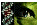Table 2 of Sultana, Mol Vis 2003; 9:730-734.

Table 2. Sequence alignment of sulfotransferases showing conservation of amino acids mutated in MCD patients

The protein sequences of the sulfotransferases shown were aligned using the software Omiga version 2.0 (Oxford Molecular Ltd., Cambridge, England). Residues mutated along with flanking amino acids are shown in this table. Mutations are indicated at the top of each column. GenBank accession numbers of the sequences are given in parentheses.

 ``` G52D, R202S, S53L S98W F107S F121L P204Q D221E/Y S210F ---------------------------- ------- ------- ------- ------- ------- ------- ------- Human corneal S G S S R S V F D V F D L F Q W V R D P A R D N L R S R GlcNAc 6-O-sulfotransferase (AF219990) Human intestinal GlcNAc S G S S R S I F D V F D F F N W V R D P A R D N L R S R 6-O-sulfotransferase (AF246718) N-acetylglucosamine- S G S S R A V F S V F D L F Q W V R D P M I D S F R S R 6-O-sulfotransferase (human) (AF131235) Human chondroitin T G S S R S L F S V L R L F R W F R D P L R E S H N S R 6-sulfotransferase-2 (AB037187) Mouse L-selectin ligand S G S S R S V F S V F D L F Q W V R D P V V D S F R S R sulfotransferase (AF131235) N-acetylglucosamine- S G S S R S V F D V F D L F Q W V R D P A R D N L R S R 6-O-sulfotransferase (Mouse) (AF176841) Mouse chondroitin T G S S K Q L L Y V L E L F R R V R D P Y E N W L A S R 6-sulfotransferase (AB008937) ```

Sultana, Mol Vis 2003; 9:730-734 <http://www.molvis.org/molvis/v9/a87/>